Introduction
Trigonometry is a Greek word meaning measuring the sides of a triangle. As a tribute to its country of origin, the Greek word has been retained to be the universal name of this subject. Trigonometry covers a very wide range of topics that are useful to us
The basic trigonometric ratios are Sine, Cosine and Tangent. With reference to a right triangle these ratios are equal to the ratios of the measures of the specific sides of the right triangles. The reciprocal ratios of Sine, Cosine and Tangent are called as Cosecant, Secant and Cotangent respectively.
Since an angle is a measure of rotation, the angles measured in counterclockwise direction are considered as positive.
A complete rotation means an angle 0f 360o or 2π radians. A complete rotation is divided into four equal sectors as quadrants 1, 2, 3 and 4 taken in the order of counterclockwise direction. All ratios are positive in quadrant 1, Sine ratio is positive in quadrant 2, Tangent ratio is positive in quadrant 3 and Cosine ratio is positive in quadrant 4.
The best way to perceive the concept and the practical applications, we will run through a tutorial of questions and answers picked up from all the important topics. For easier understanding, let us pick up questions and answers topic wise in a logical sequence. We will also give a brief explanation on the basic concepts of each topic before going through the trigonometry questions and answers.

## Trigonometry Questions and Answers

Let us begin with questions on simple concepts of trigonometric ratios.
Question 1: Given that sin x = 3/5 and cos x < 0. Find the values of all the remaining trigonometric ratios
.
It says that sin x = 3/5. That is sin x is positive. We know that the sine ratio is positive in quadrants 1 and 2 and Cosine ratio is positive in quadrants 1 and 4. Therefore, if sin x is positive and cos x is negative, then the angle 'x' lies in quadrant 2.
Let us draw a right triangle with the sine ratio of 3/5 in quadrant 3.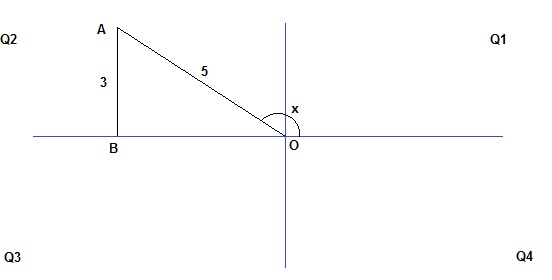OAB is a right triangle with angle 'x' in quadrant 3. Since sin x = 3/5, AB/OA = 3/5 or AB = 3 and OA = 5
Since OAB is a right triangle, OB = - √(OA2 - AB2) =  - √(52 - 32) = - √16 = -4 (The negative root is taken because in Q2 the horizontal measures are negative)
Therefore, cos x = OB/OA = -4/5 and tan x = AB/OB = 3/-4 = -3/4
The reciprocal ratios are , csc x = 1/sin x = 5/3, sec x = 1/cos x = -5/4 and and cot x = -4/3

Question 2: A flag post is tied by a stay wire from its top to a point on the ground 40 ft from the foot of the post. The wire makes an angle of 300 with the ground. Find the length of the wire and the height of the flag post. (Express your answer in radical form.)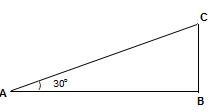In the above diagram, BC is the flag post, AC is the stay wire. AB is given as 40 ft.
AB/ AC = cos 30o = √3/2 (from tables). Hence AC = (2/√3)(AB) = (80√3)/3 ft.
BC/AC = sin 30o = 1/2 (from tables). Hence BC = (1/2)AC = (40√3)/3 ft.
Thus, the length of the wire is (80√3)/3 ft and the height of the flag post is (40√3)/3 ft.

One of the wonderful applications of trigonometry is the measurement of unknown distances and heights. Such measurements can be done by measuring the necessary angles and by effectively using the trigonometric ratios of such angles. The angle which is measured above the normal horizontal line of sight is called the angle of elevation and angle which is measured below the normal horizontal line of sight is called the angle of depression. The following question and answers will give an idea on applications of trigonometric ratios.
Question 3: An airplane is flying at an altitude of 40,000 ft. On the ground an observer sights the plane at an angle of elevation of 70o. After one minute, the observer at the same position finds the angle of elevation is decreased to 40o. Assuming the airplane is flying at the same altitude, find the speed of the airplane.
The following diagram shows the position of the airplane at different times.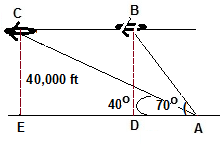In the above diagram A is the stationary position of the observer. B is the first position of the airplane and C is the position of the airplane after 1 minute.
Now, AE = CE cot 40o ≈ 40,000ft*1.19 ≈ 47670 ft and AD = BD cot 70o ≈ 40,000ft*0.364 ≈ 14559 ft
Therefore, CB = ED = AE - ED = 47670 ft - 14559 f = 33,111 ft.
The airplane covered this distance in one minute, that is, in 60 seconds.
The distance covered by the plane in one second = 33,111/60 = 551.85 ft
Therefore, the speed of the airplane = 551.85 ft/s  ≈ 376 mph.

Question 4: A light house of 60 ft height views two boats on a sea at angles of depressions of 20 deg and 50 deg. Find the distance between the two boats?
The position of the boats are shown in the following diagram.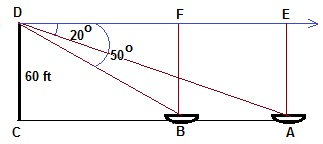Let CD be the lighthouse and A and B are the positions of the boats when the angles of depression are measured.
DE/AE = cot ADE or DE = AE cot ADE = CD cot ADE = 60 ft* cot 20 ≈ 60 ft* 2.75 = 165 ft
DF/BF = cot BDF or DF = BF cot BDF = CD cot BDF = 60 ft* cot 50 ≈ 60 ft* 0.84 = 50.4 ft
Therefore, AB = EF =DE - DF = 165 ft - 50.4 ft = 114.6 ft
Hence, the distance between the two boats is 114.6 ft

Question 5: An observer views the top of a building at an angle of elevation of 35o. After walking 50 ft towards the building he finds the angle of elevation increases to 40o. Find the height of the building. Express your answer to the nearest foot.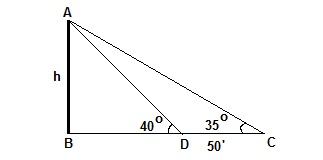In the above drawing AB represents the building, C is the first position of the observer and D is the second position of the observer. Let AB = h and CD is given as 50 ft.
From the diagram,
BC/AB = cot ACB or, BC/h = cot 35o or, BC = h cot 35o or, BC ≈ h (1.43) = 1.43h
BD/AB = cot ADB or, BD/h = cot 40o or, BD = h cot 40o or, BD ≈ h (1.19) = 1.19h
Now, DC = BC - BD or, 50 ft = 1.43h - 1.19h or, 50 ft = 0.24h
So, h = 50ft/0.24 = 208.333... ft ≈ 208 ft.
Thus, the height of the building is 208 ft.

There are many formulas and identities in trigonometry. Basically the formulas are on sum, difference, double or half of angles. They are helpful in finding the exact values (in radical form) of some non standard angles. The identities are established by use of fundamental identities related to basic trigonometry ratios. Let us try questions on these categories.Question 6: Find the exact values of tan 75o and sec 15o.
The angle 75o can be written as the sum of two principal angles as (45o + 30o)
Hence tan 75o = tan (45o + 30o)
The standard formula for tangent of sum of angles is, tan (x + y) = [tan x + tan y]/[1 - tan x tan y]
Therefore, tan 75o = tan (45o + 30o) = [tan 45o + tan 30o]/[1 - tan 45o tan 30o]
Plugging in the known values, tan 75o = [1 + (√3/3)]/[1 -  (√3/3)] = [3 + (√3)]/[3 - (√3)]
= [3 + (√3)]2/[32 - (√3)2]
= [9 + 3 + 6√3]/[9 - 3] = [12+ 6√3]/ = (2 +  √3)
Thus, tan 75o = (2 +  √3)
The angle 15o can be written as the difference of two principal angles as (45o - 30o)
Hence cos 15o = cos (45o - 30o)
The standard formula for cosine of difference of angles is, cos (x - y) = (cos x cos y + sin x sin y)
Therefore,cos15o = cos (45o - 30o) = (cos 45o cos 30o + sin 45o sin 30o)
Plugging in the known values, cos15o = [(√2/2)(√3/2) + (√2/2)(1/2)] = (√6/4 +√2/4)] = (√6 +√2)/(4)] = [√2(√3 + 1)]/6]
Thus, cos 15o = [√2(√3 + 1)]/6]

Question 7: Find the exact values of cos 75o*sin 15o
As per the product to sum formula, cos A* sin B = (1/2) [sin (A + B) - sin (A – B)]
Plugging in A = 75o and B = 15o,
cos 75o* sin 15o = (1/2) [sin (75o + 15o ) - sin (75o – 15o)] = (1/2) [ sin 90o – sin 60o]
Plugging in the known values, cos 75o* sin 15o = (1/2) [1 - (√3/2)] = (1/2) [(2 - √3)/2] = (2 - √3)/4
cos 75o*sin 15= (2 - √3)/4

Question 8: Prove the identity (cos x + sin x)/(cos x - sin x) = (1 + sin 2x)/(cos 2x)
Let us start from the left side.
Left side = (cos x + sin x)/(cos x - sin x)
= [(cos x + sin x)(cos x + sin x)]/[(cos x - sin x)(cos x + sin x)]
=  (cos x + sin x)2/ (cos2 x - sin2 x)
= [cos2 x + sin2 x + 2sin x* cos x]/ (cos2 x - sin2 x)
= [1 + 2sinx* cos x]/ (cos2 x - sin2 x)    since, cos2 x + sin2 x = 1
= (1 + sin 2x)/(cos 2x)    since, 2sinx* cos x = sin 2x and cos2 x - sin2 x = cos 2x
Thus, (cos x + sin x)/(cos x - sin x) = (1 + sin 2x)/(cos 2x)

There are two important laws in trigonometry with which you can completely solve a triangle. That is, with minimal details of measures of angles and measures of sides of a triangle, you can figure out all the remaining measures of angles and sides. These laws are called law of sine and law of cosine. Let us try to understand these laws from the following trigonometric questions and answers.

Question 9: In a triangle ABC, the measures of the sides are a = 15 cm, b = 25 cm and c = 30 cm. Find the measures of all the angles.
We are aware of only the measures of all the sides but not aware of the measure of any of the angles. In such a case, the cosine law helps us to find any one of the angles. Let us try to find the measure of angle C. As per cosine law,
c2 = b2 + a2 - 2ba cos C
or, cos C = (b2 + a2 - c2 )/(2ba)
Plugging in the given values, cos C = [(25 cm)2 + (15 cm)2 - (30 cm)2 )]/[(2)(25 cm)(15 cm)] = [625 + 225 - 900]/ = -50/750 = -1/25 = -0.04
or, C = cos -1 (-0.04) ≈ 92.3o.
Now it is easier to use law of sine to find the remaining angles. Let us next fine angle B. As per sine law,
[(sin B) / b] = [(sin C) / c ] or, sin B = [b * sin C] / [c]
So, sin B = [25 cm * sin 92.3o] / [30 cm] ≈ [5 * 0.999]/ ≈ 0.837
Hence, B = sin-1 0.837 ≈ 56.4o
The remaining angle can be found by using triangle angles sum identity. That is,
A = 180o - (B + C) = 180o - (56.4o + 92.3o) = 180o - 148.7o
or,  A = 31.3o

Question 10: Explain how to determine the type of a triangle without knowing the measure of any of its interior angles.
The type of a triangle can be determined without knowing the measure of any of its interior angles by using cosine law of triangles. Let ABC be any scalene triangle and let 'a', 'b' and 'c' are the measures of corresponding sides with 'c' being the largest.In such a case, angle C is the largest angle. As per law of cosine,
c2 = b2 + a2 - 2ba cos C
or, cos C = (b2 + a2 - c2 )/(2ba)
The denominator of the right side is always positive. Therefore,
if, b2 + a2 > c2 , cos C is > 0, means angle C is acute angle. Hence the triangle is an acute triangle.
if, b2 + a2 = c2 , cos C is = 0, means angle C is right angle. Hence the triangle is a right triangle.
if, b2 + a2 < c2 , cos C is < 0, means angle C is obtuse. Hence the triangle is an obtuse triangle.